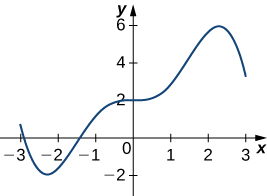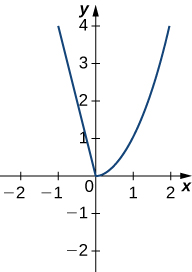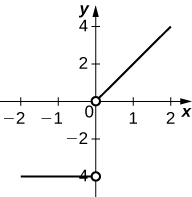True or False ? Justify the answer with a proof or a counterexample.

1.  Every function has a derivative.

#### Solution

False. Counterexample: $f(x) = |x|$

2.  A continuous function has a continuous derivative.

3.  A continuous function has a derivative.

#### Solution

False. Counterexample: $f(x) = |x|$

4.  If a function is differentiable, it is continuous.

Use the limit definition of the derivative to exactly evaluate the derivative.

5.  $f(x)=\sqrt{x+4}$

#### Solution

$f'(x) =\frac{1}{2\sqrt{x+4}}$

6.  $f(x)=\frac{3}{x}$

7. $f(x)=\frac{9}{x^2}$

#### Solution

$f'(x) = -\frac{18}{x^3}$

8. $f(x)=\sqrt{3x+1}$

Find the first derivative of the following functions.

9.  $f(x)=3x^3-\frac{4}{x^2} + \pi^6$

#### Solution

$f'(x) = 9x^2+\frac{8}{x^3}$

10.  $f(x)=(4-x^2)^3$

11.  $f(x)=e^{\sin x}$

#### Solution

$f'(x) = e^{\sin x} \cos x$

12.  $f(x)=\ln(3x^8+2)$

13.  $g(x)=x^2 \cos x+x \tan x$

#### Solution

$g'(x) =x \sec^2 x+2x \cos x+ \tan x-x^2 \sin x$

14.  $f(x)=(\sqrt{3x^2+2})(e^{3x} - \ln |x|)$

15.  $f(x)=\frac{x^2 + \sin x}{2+ \tan x}$

#### Solution

$f'(x) =\frac{(2x + \cos x)(2+ \tan x) - \sec ^2 x(x^2 + \sin x)}{(2+\tan x)^2}$

16. $y= (\sec (2x) + x^3 + e^x)^{99}$

17. $v(t)= t^2 - \frac{1}{\sqrt{t}^6}$

#### Solution

$v'(t) = 2t + \frac{6}{5} t^{-\frac{11}{5}}$

18. $f(x) = \sqrt{2x + \sqrt{3x}}$

19. $f(\theta)= \tan(\cos (3\theta ^2 +1))$

#### Solution

$f'(\theta) = -6 \theta \sec ^2 (\cos (3 \theta ^2+1) \sin (3 \theta ^2+1)$

20. $f(x)= \log_7 (2x +e^{-x})$

21. $f(x)= \ln |9x^3 - e^{2x} + 5^{x}|$

#### Solution

$f'(x) = \frac{27x^2 -2e^{2x} + 5^x \ln 5}{9x^3 - e^{2x} + 5^{x}}$

22. $f(x)=6^{3x^2 -e^6 + x^\pi}$

23. $f(x)= \log_6 (2x^7 - e^{x^5 -\pi x} + \sin x)$

#### Solution

$f'(x) = \frac{14x^6 - e^{x^5 -\pi x} (5x^4 - \pi) + \cos x }{\ln 6 (2x^7 - e^{x^5 -\pi x} + \sin x)}$

24. $g(x)= \log_2 [\ln (4x^6 -3x + 1]$

25. $2xy = y^2 + 6$

#### Solution

$y' = \frac{y}{y-x}$

26.  $x^2 y=(y+2)+xy \sin (x)$

27. $f(x) = x^{2x}$

#### Solution

$f'(x) = 2x^{2x}(\ln x +1)$

28. $f(x) = 6x^{\sin x}$

29. $f(x) = x^4 + x^{\cos x}$

#### Solution

$f'(x) = 4x^3 +x^{\cos x}(-\sin x \ln x + \frac{\cos x}{x})$

30. $f(x) = x^2 + x^{\sin x}$

Find the following derivatives of various orders.

31.  First derivative of $y=x \ln x \cos x$

#### Solution

$y' = \cos x (\ln x+1) -x \ln x \sin x$

32.  Third derivative of $y=(3x+2)^2$

33.  Second derivative of $y=4^x+x^2 \sin x$

#### Solution

$y'' = 4^x(\ln 4)^2+2 \sin x+4x \cos x-x^2 \sin x$

34.  The 27th derivative of $2x^{16} + e^{3x} +\cos x$

35.  The 38th derivative of $f(x) = 2x^{22} + e^{2x} +\cos x$

#### Solution

$f^{(38)} (x) = 0 + 2^{38} e^{2x}-\cos x$

Find the equation of the tangent line to the following equations at the specified point.

36.  $y= \cos x+x$ at $x=0$

37.  $y=x+e^x-\frac{1}{x}$ at $x=1$

#### Solution

$y=(2+e)x-2$

38. $x^2y^3 - x^3 = 3y^2-7$ at the point $(2,1)$

39. $f(x) = (x+2)^{x^2}$ at $x=-1$

#### Solution

$y = x+ 2$

Determine the following limits.

40. $\underset{x\to 0}{\lim} \frac{\tan x}{6x}$

41. $\underset{x\to 0}{\lim} \frac{\sin x}{x + \tan x}$

#### Solution

$\frac{1}{2}$

42. $\underset{\theta \to 0}{\lim} \frac{1-\cos ^2 \theta}{\sin \theta}$

43. $\underset{x\to 2}{\lim} \frac{\sin (x-2)}{x^2+2x-8}$

#### Solution

$\frac{1}{6}$

Draw the derivative for the following graphs.

44.45.#### Solution46. A particle moves on a vertical line so that its coordinate at time $t$ is $y= t^3 -18t+7, t \ge 0$.

a) Find the velocity function, $v(t)$.
b) Find the acceleration function, $a(t)$.
c) When is the particle moving upward? When is the particle moving downward?

47. A ball is thrown vertically in the air with an upward velocity of 80 ft per second. Its height after $t$ seconds is $h = 80t - 10t^2$.

a) What is the maximum height reached by the ball? How many seconds does it take for the ball to reach its maximum height?
b) Find the velocity function, $v(t)$.
c) What is the velocity of the ball at $t = 3$?
d) What is the velocity of the ball $t = 5$?
e) How long does it take for the ball to reach the ground?

#### Solution

a) 160 ft; 4 seconds
b) $v(t) = 80 - 20t$
c) $v(3) = 80 - 20 (3) = 20$ ft/s (upward)
d) $v(5) = 80 - 20 (5) = -20$ ft/s (downward)
e) $0 = 80t - 10t^2$ so $t=8$ seconds

The following questions concern the water level in Ocean City, New Jersey, in January, which can be approximated by $w(t)=1.9+2.9 \cos (\frac{\pi}{6}t)$, where $t$ is measured in hours after midnight, and the height is measured in feet.

48.  Find and graph the derivative. What is the physical meaning?

49.  Find $w^{\prime}(3)$. What is the physical meaning of this value?

#### Solution

$w^{\prime}(3)=-\frac{2.9\pi}{6}$. At 3 a.m. the tide is decreasing at a rate of 1.514 ft/hr.

The following questions consider the wind speeds of Hurricane Katrina, which affected New Orleans, Louisiana, in August 2005. The data are displayed in a table.

Wind Speeds of Hurricane Katrina
Source: http://news.nationalgeographic.com/news/2005/09/0914_050914_katrina_timeline.html.
Hours after Midnight, August 26 Wind Speed (mph)
1 45
5 75
11 100
29 115
49 145
58 175
73 155
81 125
85 95
107 35

50.  Using the table, estimate the derivative of the wind speed at hour 39. What is the physical meaning?

51.  Estimate the derivative of the wind speed at hour 83. What is the physical meaning?

#### Solution

-7.5. The wind speed is decreasing at a rate of 7.5 mph/hr